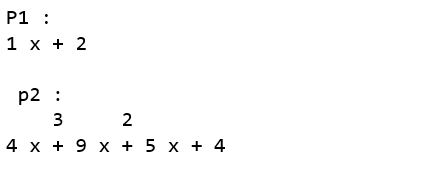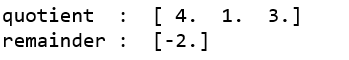numpy.polydiv() in Python

• Last Updated : 04 Dec, 2020

The numpy.polydiv() method evaluates the division of two polynomials and returns the quotient and remainder of the polynomial division.

Syntax : numpy.polydiv(p1, p2)
Parameters :
p1 : [array_like or poly1D]Coefficients of dividend polynomial.
p2 : [array_like or poly1D]Coefficients of divisor polynomial.

Return:
q : [ndarray]Coefficients of quotient.
r : [ndarray]Coefficients of remainder.

Code : Python code explaining polydiv()

 # Python code explaining # numpy.polydiv()    # importing librariesimport numpy as npimport pandas as pd  # Constructing polynomial p1 = np.poly1d([1, 2]) p2 = np.poly1d([4, 9, 5, 4])     print ("P1 : ", p1) print ("\n p2 : \n", p2)quotient, remainder = np.polydiv(p2, p1)  print("\n\nquotient  : ", quotient)print("remainder : ", remainder)print ("\n")# Defining ndarrayx = np.array([1, 2])y = np.array([4, 9, 5, 4])  quotient, remainder = np.polydiv(y, x)  print("quotient  : ", quotient)print("remainder : ", remainder)Attention geek! Strengthen your foundations with the Python Programming Foundation Course and learn the basics.

To begin with, your interview preparations Enhance your Data Structures concepts with the Python DS Course. And to begin with your Machine Learning Journey, join the Machine Learning - Basic Level Course

My Personal Notes arrow_drop_up